# Revealing#

Presentations often contain slides that are revealed gradually in several steps (usually called fragments). Elsie allows you to create multiple fragments per slide and selectively show individual boxes in specific fragments using the `show` parameter.

Fragments on a slide are counted from `1` and there is always at least a single fragment in each slide. The number of fragments of a slide is determined by the highest fragment number in which some box is visible.

To display a box in a specific fragment, pass the fragment number as a string to the `show` parameter:

``````slide.box().text("Box 1")
slide.box(show="2").text("Box 2")
``````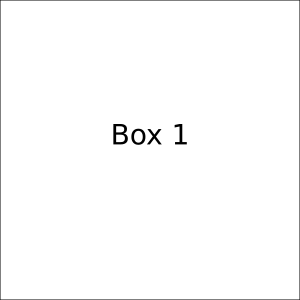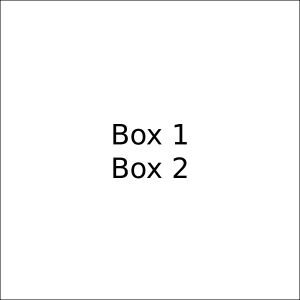Fragment: 1

The slide above has two fragments, because the second box will be shown in fragment `2`. You can use the buttons below the slide to move between individual fragments.

Fragment visibility is hierarchical, i.e. if you hide a parent, all of its children will be hidden as well:

``````box = slide.box(show="2")
box.box().text("Box 1")
box.box().text("Box 2")
``````Fragment: 1

## Fragment selectors#

In addition to choosing a single specific fragment in which should a box be displayed, there are other values that you can pass to the `show` parameter:

• `None` (default): The box will be shown in all steps. This is equivalent to passing `"1+"`.
• `"<number>"`: The box will be shown only in the fragment with the given number.
• `"<number>+"`: The box will be shown in the fragment with the given number and also in all following fragments. This is called an open fragment range.
• `"<from>-<to>"`: The box will be shown in fragment `from` and it will stay shown until fragment `to`. This is called a closed fragment range. The range is inclusive from both sides.

Here is an example of the various `show` values in action:

``````slide.box().text("Box 1")
slide.box(show="2-3").text("Box 2")
slide.box(show="2+").text("Box 3")
slide.box(show="4").text("Box 4")
``````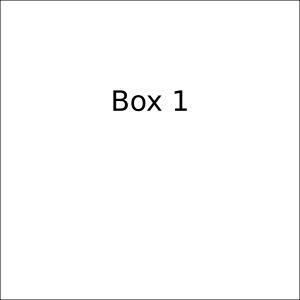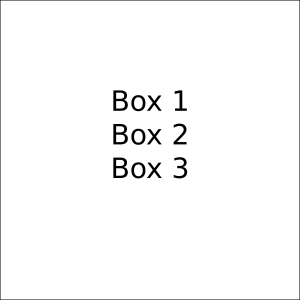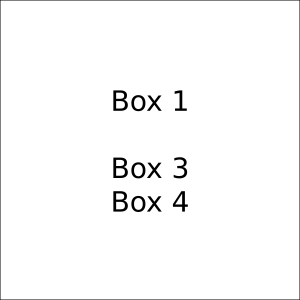Fragment: 1

The first box will be shown in all fragments, the second box in fragments `2` and `3`, the third box in all fragments from `2` to the end, and the fourth box will be shown only in fragment `4`. The largest fragment number present in the slide is `4`, so the slide will have four fragments in total.

## Fragment placeholders#

If you have a lot of fragments on a slide, it might be tedious to count the fragment numbers manually and keep them in sync when you make changes to the slide. To make this process easier, you can use placeholders to refer to the current fragment.

The current fragment is the fragment with the largest number defined on a slide so far. You can access its number using the `current_fragment` method. This value changes dynamically as you add more fragments to the slide:

``````# slide.current_fragment() == 1
slide.box().text("Box 1")
# slide.current_fragment() == 1
slide.box(show="2").text("Box 1")
# slide.current_fragment() == 2
slide.box(show="3").text("Box 1")
# slide.current_fragment() == 3
``````

To leverage the current fragment, you can use two placeholders in place of a fragment number passed to `show`:

• The `"last"` placeholder resolves to the value of the current fragment.
• The `"next"` placeholder increments the current fragment and then resolves to the incremented value.

Using the placeholders, you can show boxes in the same order as they appear in the source code. Here is an example of their usage:

``````slide.box().text("Box 1")

# Moves current fragment to 2 and shows the box in fragment 2
slide.box(show="next").text("Box 2")

# Shows the box in fragment 2
slide.box(show="last").text("Box 3")

# Moves current fragment to 3 and shows the box in fragment 3
slide.box(show="next").text("Box 4")
``````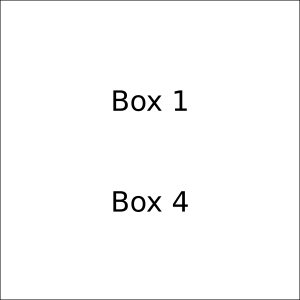Fragment: 1

You can also combine the placeholders with open fragment ranges:

``````slide.box().text("List item 1")
slide.box(show="next+").text("List item 2")
slide.box(show="last+", p_left=20).text("Subitem 1")
slide.box(show="last+", p_left=20).text("Subitem 2")
slide.box(show="next+").text("List item 3")
slide.box(show="last+", p_left=20).text("Subitem 1")
``````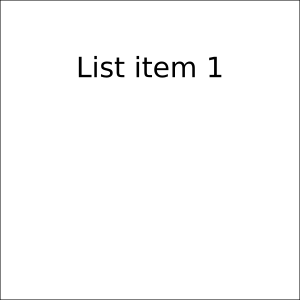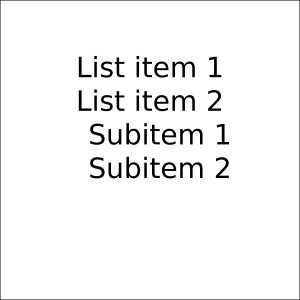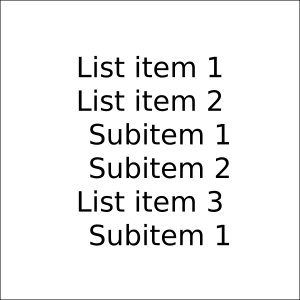Fragment: 1

## Overlaying boxes#

As you may have already noticed, using `show` only influences the visibility of a box, not its layout. Therefore, the space for a box is allocated in each fragment, even if the box is not visible in that fragment (if you know CSS, fragment visibility behaves like `visibility: hidden`, not `display: none`).

If you instead want to show boxes gradually on top of one another, you can use the `overlay` shortcut to render a box at the same location and size as another box.

``````slide.overlay(show="1").text("Box 1")
slide.overlay(show="2").text("Box 2")
slide.overlay(show="3").text("Box 3")
``````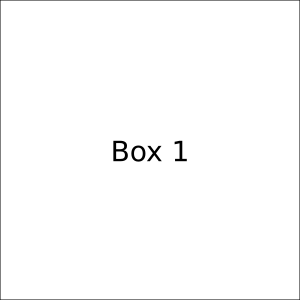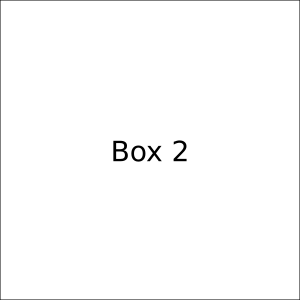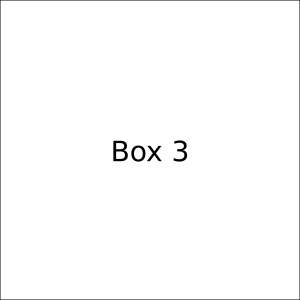Fragment: 1

When using overlays, make sure that the box that you are overlaying is actually visible in the fragment when you want it to be overlaid. For example, this may not work as expected:

``````box = slide.box(show="1").text("Box 1")
box.overlay(show="2").text("Box 2")
box.overlay(show="3").text("Box 3")
``````Fragment: 1

Because boxes `2` and `3` are children of box `1`, which is only shown in fragment `1`.